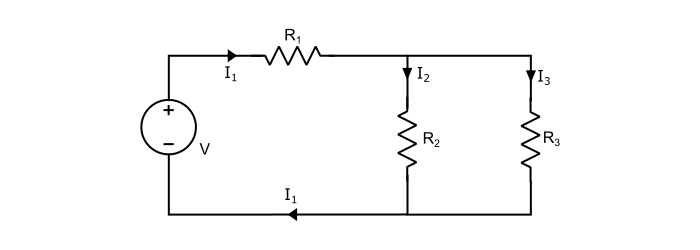# Series-Parallel Circuit: Definition and Examples

A series-parallel circuitis a combination of series and parallel circuits. In this circuit some of the elements are connected in series fashion and some are in parallel.

In the circuit shown below, we can see that resistors R2 and R3 are connected in parallel with each other and that both are connected in series with R1.To solve such circuits, first reduce the parallel branches to an equivalent series branch and then solve the circuit as a simple series circuit.

Here, RP is equivalent resistance of parallel combination given by,

$$\mathrm{\mathit{R}_{p}=\frac{\mathit{R}_{2}\mathit{R}_{3}}{\mathit{R}_{2}+\mathit{R}_{3}}}$$

Total circuit resistance (RT) is given by,

$$\mathrm{\mathit{R}_{r}=\mathit{R}_{1}+\mathit{R}_{p}=\mathit{R}_{1}+\frac{\mathit{R}_{2}\mathit{R}_{3}}{\mathit{R}_{2}+\mathit{R}_{3}}}$$

Voltage across the parallel combination is given by,

$$\mathrm{\mathit{V}_{p}=\mathit{I}_{1}\mathit{R}_{p}=\mathit{I}_{1}×\frac{\mathit{R}_{2}\mathit{R}_{3}}{\mathit{R}_{2}+\mathit{R}_{3}}}$$

The total power dissipated in the circuit is equal to the sum of powers dissipated in the individual resistances, as,

$$\mathrm{\mathit{P}_{r}=\mathit{I}_{1}^{2}\mathit{R}_{1}+\mathit{I}_{2}^{2}\mathit{R}_{2}+\mathit{I}_{3}^{2}\mathit{R}_{3}}$$

## Numerical Example

In the circuit shown below, find the value of unknown resistance R, also determine the total resistance of the circuit and total power dissipated. It is given that voltmeter shows a reading of 10 V.Solution

Referring to the circuit shown above,

Total resistance of the parallel combination,

$$\mathrm{\mathit{R}_{p}=\frac{600 × 100}{600 + 100}=85.71\:Ω}$$

As, the voltage across the parallel combination is equal to voltmeter reading that is 10 V (given). Therefore,

$$\mathrm{Circuit\:current\:\mathit{I}=\frac{10}{85.71}=0.1167\:A}$$

$$\mathrm{Voltage\:across\:\mathit{R}=24 − 10 = 14\:V}$$

Hence,

$$\mathrm{Value\:of\:\:\mathit{R}=\frac{14}{0.1167}=119.96\:Ω}$$

Total resistance of the circuit is,

$$\mathrm{\mathit{R}_{r}=\mathit{R}_{p}+\mathit{R}=119.96 + 85.71 = 205.67\:Ω}$$

Total power dissipated in the circuit is,

$$\mathrm{\mathit{P}_{r}=\mathit{I}^{2}\mathit{R}_{r}=(0.1167)^{2}× 205.67 = 2.8\:W}$$